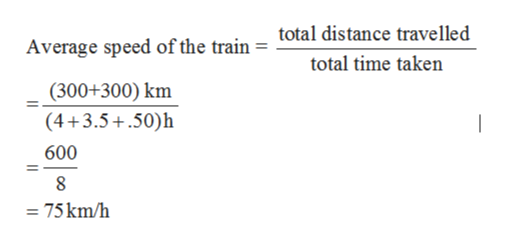# A train makes a round trip on a straight, level track. The first half of the trip is 300km and is traveled at a speed of 75 km/m. After a 0.50 h layover, the train returns the 300km at the speed of 85 km/h. What is the train’s (a) average speed and (b) average velocity?

Question
22 views

A train makes a round trip on a straight, level track. The first half of the trip is 300km and is traveled at a speed of 75 km/m. After a 0.50 h layover, the train returns the 300km at the speed of 85 km/h. What is the train’s (a) average speed and (b) average velocity?

check_circle

Step 1

Given:

For first half of the trip

Distance covered by train s1 = 300 km

Speed of the train v1 = 75 km/h (assumed)

Layover time of the train = 0.50 h

Time taken by the train during its first trip

Step 2

For second half of the trip

Distance covered by train s2 = 300 km

Speed of the train v2 = 85 km/h

Time taken by the train during its second trip

Step 3

(a) Average speed of the trai...help_outlineImage Transcriptionclosetotal distance travelled Average speed of the train total time taken (300+300) km (4+3.5 .50)h 600 8 75km/h fullscreen

### Want to see the full answer?

See Solution

#### Want to see this answer and more?

Solutions are written by subject experts who are available 24/7. Questions are typically answered within 1 hour.*

See Solution
*Response times may vary by subject and question.
Tagged in

### Physics